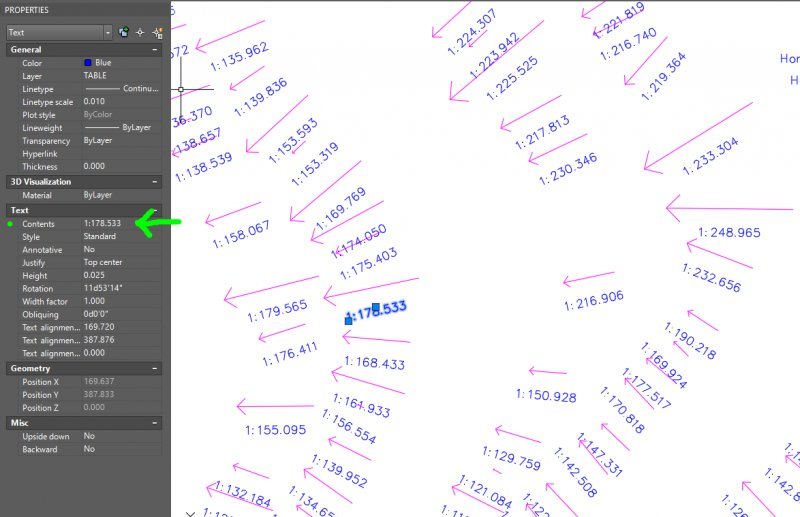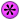# Need LISP Function to read text contents and convert ratio to number.## Recommended PostsI have a vector map of a floor and all i am given are vectors and grade ratios (i.e. 1:155.095, 1:97.561) of the floor. The problem is that I only want to look at the areas of the floor that are below a certain grade ratio (1:69), but they are ratios set as Text Contents so AutoCAD doesnt understand that these ratios are numbers.

I am looking for a Lisp function that, for each text if a ratio across an entire floor (or the layout), copy the text (which is a ratio), find out the number that the ratio is (i.e. take the 1 and divide by 69), then replace the text with just the number (0.0145). I want this to do this for every instance, and there are over 37,000 of these texts on the floor in question.

I have attached an image that depicts what I have in the layout.

Does anyone know of a lisp function that can do this?##### Share on other sitesQuickly written:

```(defun c:fixratios ( / a b e i p s x )
(if (setq s (ssget "_:L" '((0 . "TEXT")(1 . "#*:#*")(1 . "~*[~.:0-9]*")(1 . "~**"))))
(repeat (setq i (sslength s))
(setq e (entget (ssname s (setq i (1- i))))
x (cdr (assoc 1 e))
p (vl-string-position 58 x)
)
(if (and (setq a (distof (substr x 1 p) 2))
(setq b (distof (substr x (+ 2 p)) 2))
(not (equal 0.0 b 1e-)
)
(entmod (subst (cons 1 (rtos (/ a b))) (assoc 1 e) e))
)
)
)
(princ)
)```

##### Share on other sitesHi Lee needs a second part say a copy of the text onto a different layer where the result is

had a little bit of time.

```; original code by Lee-mac Aug 2017
; changed to only modify 1:x below 1:69
; by Alan H Aug 2017

(defun c:fixratios ( / a b e i p s x ab)
(if (/= (tblsearch "Layer" "Xgrade") nil)
)

(if (setq s (ssget "_:L" '((0 . "TEXT")(1 . "#*:#*")(1 . "~*[~.:0-9]*")(1 . "~**"))))
(repeat (setq i (sslength s))
(setq e (vlax-ename->vla-object (ssname s (setq i (1- i)))))
(setq x (vla-get-textstring e))
(setq p (vl-string-position 58 x))
(if (and
(setq a (distof (substr x 1 p) 2))
(setq b (distof (substr x (+ 2 p)) 2))
(not (equal 0.0 b 1e-)
(< (setq ab (/ a b)) (/ 1.0 69.0))
)
(progn
(vla-put-textstring  e (rtos ab 2 3))
)
)
)
)
(princ)
)
```

Edited by BIGAL
##### Share on other sitesHi lee , nice lisp.

Could you explain

`(setq s (ssget "_:L" '((0 . "TEXT")(1 . "#*:#*")(1 . "~*[~.:0-9]*")(1 . "~**"))))`

Also it can be add the 8 dxf code to TABLE , as it is the text's layer.

##### Share on other sitesCould you explain

`(setq s (ssget "_:L" '((0 . "TEXT")(1 . "#*:#*")(1 . "~*[~.:0-9]*")(1 . "~**"))))`

(1 . "#*:#*")

Matches text starting with a number and containing a colon followed by a number, e.g. "1abc:2def"

(1 . "~*[~.:0-9]*")

Matches text which only contains the characters ".:0123456789", e.g. "1.23:4.56:7.89"

[noparse](1 . "~**")[/noparse]

Ensures that the string only contains one colon.

This doesn't account for all cases, as you could perform additional validation on the decimal point (ensuring it appears at most once on either side of the colon).

The layer filter could also be included, but it would reduce the ability for other members to easily use the code.

## Join the conversation

You can post now and register later. If you have an account, sign in now to post with your account.
Note: Your post will require moderator approval before it will be visible.×   Pasted as rich text.   Restore formatting

Only 75 emoji are allowed.